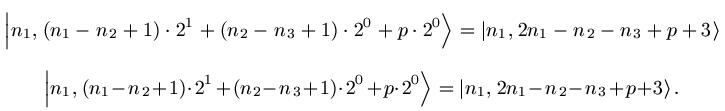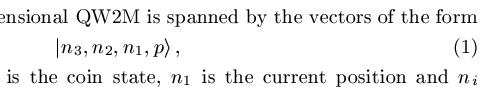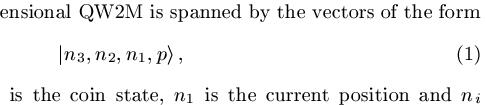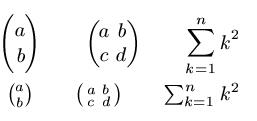## Latex公式压缩

2019-05-05 18:05  chloe_zhou  阅读(9761)  评论(0编辑  收藏  举报

序言

在用Latex进行公式排版时，容易出现公式长度超过一行或者公式上下占用较大文本区域的情况。对此我们可以从多个方面对其进行调整，以压缩公式所占据的面积，从而有助于实现自己需要的排版效果。

正文

1. 缩小公式内部的空隙宽度

如果一个公式的宽度仅少量超出文本宽度，则可以通过命令"\!"压缩公式中字符之间的间距。例如由Latex代码

\begin{equation*}
\Ket{n_1,(n_1-n_2+1)\cdot 2^1+(n_2-n_3+1)\cdot 2^0+p\cdot 2^0}=\Ket{n_1,2n_1-n_2-n_3+p+3}
\end{equation*}
\begin{equation*}
\Ket{n_1,(n_1\!-\!n_2\!+\!1)\!\cdot\!2^1\!+\!(n_2\!-\!n_3\!+\!1)\!\cdot\!2^0\!+\!p\!\cdot\!2^0}    =\Ket{n_1,2n_1\!-\!n_2\!-\!n_3\!+\!p\!+\!3}\label{eq:4}.
\end{equation*}2. 缩小公式上下的空隙高度

如果感觉公式上下距离环境文本的间隙过大，则可以通过命令“\setlength\abovedisplayskip{3pt}” 和 “\setlength\belowdisplayskip{3pt}”来分别设置公式上和下的空隙高度，其中"3pt"即为空隙高度的具体数值，值越小则空隙越小。

例如代码

\begin{equation}
\setlength\abovedisplayskip{3pt}%shrink space
\setlength\belowdisplayskip{3pt}
\Ket{n_3,n_2,n_1,p}\label{eq:1},
\end{equation}

\begin{equation}
\Ket{n_3,n_2,n_1,p}\label{eq:1},
\end{equation}3. 将公式缩小为行内形式

二项式系数、矩阵、累加等符号都可以缩小为行内形式。例如代码

\begin{eqnarray*}
\end{eqnarray*}